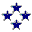Spring Counterweights on Telescopes
 Cruxis Home > Spring Counterweights The 1100 mm Cruxis Telescope

This page discusses the implementation of spring counterweights on Dobsonian telescopes, and presents an Excel sheet for computing the optimum spring properties for a particular configuration. An actual example of a bungee cord counterweight on a 16" f/5 scope is shown in some detail.

I wrote this article back in June 2009, but never came to publish it. The contents still appears relevant and useful in October 2020. So here we go!

## Introduction to Spring Counterweights

With the evergrowing number of useful telescope accessories like 2" eyepieces, barlows, coma correctors, binoviewers, filter slides or video cameras, keeping the telescope's Optical Tube Assembly (OTA) balanced can become a problem. On Dobsonian telescopes the heavy accessories near the eyepiece can make the OTA drop when observing at low altitudes, or can hinder the telescope's movements. When pushing the tube up you have to overcome the imbalance before the telescope starts moving, which can result in jerky displacements.

The standard solution for top-heavy telescopes is to add counterweights at the bottom of the OTA, near the primary mirror. Unfortunately every pound added at the eyepiece needs to be compensated by a much larger weight at the mirror box. In typical Dobsonian telescopes a 1 kg binoviewer requires about 4 kg of counterweight at the bottom.

A surprisingly effective alternative is to add one or several springs to keep the OTA balanced, a so-called "virtual counterweight". With some optimization of the spring properties one can produce designs that behave nearly exactly like an actual counterweight... but without the added mass!

Spring counterweights are affordable and easy to install - especially if you use bungee cord as spring. Bungee cords are readily available in most hardware stores and are perfect for this use: cheap, light and replaceable. You can change the "weight" of the virtual counterweight by selecting a bungee cord with different stiffness.

## A Bungee Cord Counterweight on a 16" Dobsonian

A picture of a home-made 16" f/5 telescope with spring counterweight. The solution consists of a 6 mm bungee cord about 750 mm long, two 32 mm pulleys and 3 stainless bolts with lock nuts that can be found at any hardware store at a total cost below 5 Euro.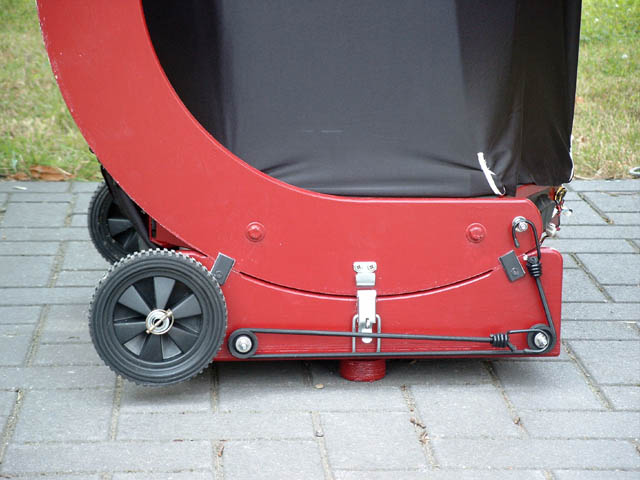The ingredients: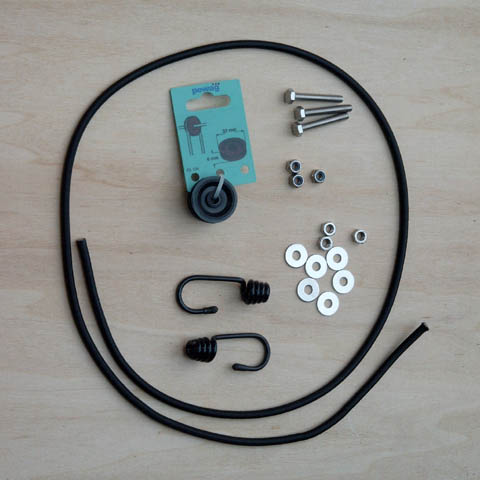The bungee cord is attached to a bolt on the altitude trunion and runs via the two pulley wheels to an attachment bolt on the rocker box. The second wheel is only required to give sufficient length.

When the telescope is lowered, the bungee cord is stretched and pulls at the mirror box.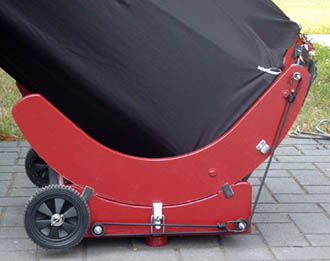Does this system really mimic a normal counterweight from zenith to horizon?
Let's find out by analysis!

## Theoretical analysis

The force and rotation moment (force multiplied by perpendicular distance) that act on the altitude trunion can be computed with some basic trigonometry.

Input data
See the image for the following items:
• C = center of the altitude trunion
• A = attachment point on rocker bock: radius RA (mm) from the center C of the altitude trunion
• B = attachment point on mirror box or altitude trunion: radius RB (mm), angle θ (degrees) from the line CA
• L0 = spring pretension length (mm) when the telescope is vertical (pointing at the zenith)
• k = spring constant (N/mm) of the bungee cord, see belowFormulae
With some trigonometry one can derive the following:
• LAB = distance between A and B = Sqrt(RA2 + RB2 - 2 RA RB cos θ)
• F = spring force = k (LAB - LAB0 + L0)
with LAB0 the distance between A and B when the telescope is vertical (pointing at the zenith)
• M = moment on mirror box = F (RA RB / LAB) sin θ
With these simple formulae it's possible to compute the rotation moment (force multiplied by distance) exerted by the bungee cord on the mirror box in several telescope positions. The angle θ varies from an initial value θ0 measured when the telescopes is vertical to the value θ0+90° when the telescope is horizontal.
The rotation moment should be as close as possible to the moment exerted by a real counterweight at the back of the mirror (to compensate for a real weight at the eyepiece), which varies with the sine of the zenith angle.
Results for 16" telescope
I have coded the formulae in an Excel sheet, using the 16" Dobsonian configuration shown above as an example. My goal was to find the best spring constant and pretension length to balance a 1 kg weight (10 N force) at the eyepiece, say, a typical binoviewer configuration. The eyepiece is at 1400 mm distance from the center of rotation of the OTA (center of the altitude trunions).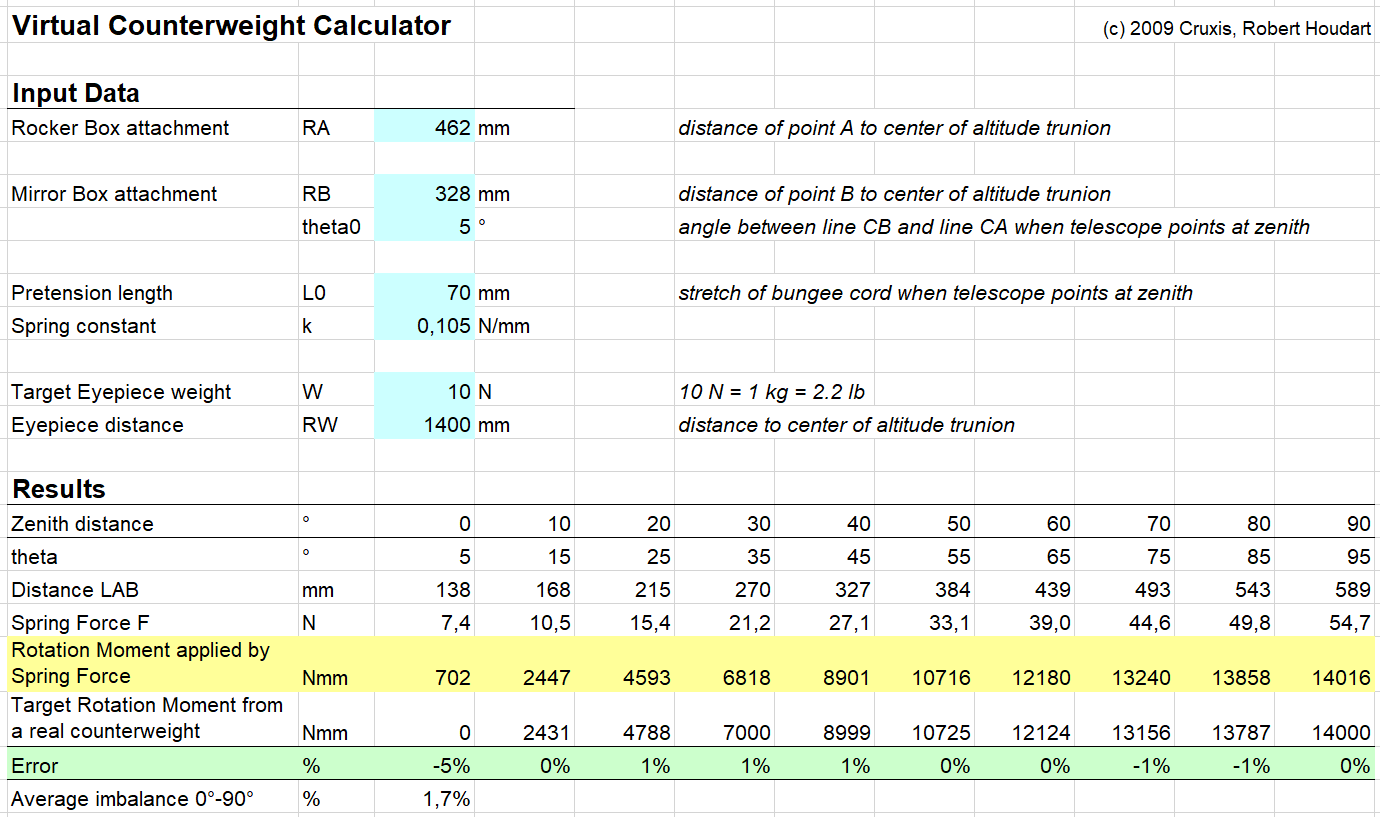Playing around with the values it appears that the best results are obtained with a pretension length of about 70 mm. The spring force will then increase from 7 N (1.5 lb) when pointing at zenith to 55 N (12 lb) when pointing at the horizon.
The maximum difference between the action of the spring and the force of a real counterweight at the back of the mirror box - the "Error" in the Excel sheet - appears in the zenith and amounts to 5% of the original load (0.05 kg at the eyepiece). Between 10° and 90° zenith distance the error is less than 2%. Quite amazing how well the spring in this particular configuration mimics an actual weight!
It is interesting to note that the optimal pretension length doesn't depend on the design load, and is effectively a constant for the particular configuration of the telescope and the attachment points A and B. In the example above, for a 1 kg eyepiece load the required spring stiffness is 0.105 N/mm and the optimal pretension length is 70 mm. For a 2 kg eyepiece load the spring stiffness would be doubled to 0.21 N/mm, but the optimal pretension length remains the same.

## Determining experimentally the bungee cord spring constant

It's very easy to measure the spring constant of your bungee cord. Hang an object of known weight at the end of your bungee cord and measure the elastic elongation. The stiffness is the ratio between weight and elongation.

For the bungee cord of the 16" telescope I used two boxes of milk (2.1 kg mass = 20.6 N weight) and measured the displacement as 280 mm. Spring stiffness is then 20.6 / 280 = 0.075 N/mm.

The Excel sheet above shows that a spring stiffness of 0.105 N/mm would balance a weight of 1 kg at the eyepiece, so this spring should be the equivalent of 0.075/0.105 * 1 kg = 0.7 kg at the eyepiece.

The actual 16" scope uses a pretension length of 80 mm - very close to the optimum value of 70 mm.

## Calculator for computing optimum spring properties

You can download the Excel sheet shown above. Adapt the position of your attachment points, select which load you want to compensate at the eyepiece, then experiment with the spring pretension length and spring constant to find the solution that is the best match.

## Some further thoughts

Ideally you should install a spring counterweight on both sides of the rocker box, so that the resulting forces are perfectly symmetrical. In practice having the spring only at one side works well also.

It's very easy to modify the virtual "counterweight" of the system by using a different bungee cord. If you plan on using heavier equipment near the eyepiece, just switch the cord (takes less than 30 seconds) and you're set. I usually carry a somewhat thicker/stiffer bungee cord when I intend to use a binoviewer. This bungee cord is actually equivalent to 6 kg of counterweight at the back of the scope!

The versatility, ease of installation and low cost of this system are truly great features. It simply works!